# Eigenvalues and eigenvectors

Eigenvalues and eigenvectors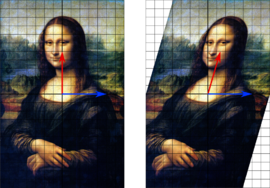In this shear mapping the red arrow changes direction but the blue arrow does not. Therefore the blue arrow is an eigenvector, with eigenvalue 1 as its length is unchanged.

The eigenvectors of a square matrix are the non-zero vectors that, after being multiplied by the matrix, remain parallel to the original vector. For each eigenvector, the corresponding eigenvalue is the factor by which the eigenvector is scaled when multiplied by the matrix. The prefix eigen- is adopted from the German word "eigen" for "own" in the sense of a characteristic description. The eigenvectors are sometimes also called characteristic vectors. Similarly, the eigenvalues are also known as characteristic values.

The mathematical expression of this idea is as follows: if A is a square matrix, a non-zero vector v is an eigenvector of A if there is a scalar λ (lambda) such that$A\mathbf{v} = \lambda \mathbf{v} \, .$

The scalar λ (lambda) is said to be the eigenvalue of A corresponding to v. An eigenspace of A is the set of all eigenvectors with the same eigenvalue together with the zero vector. However, the zero vector is not an eigenvector.

These ideas often are extended to more general situations, where scalars are elements of any field, vectors are elements of any vector space, and linear transformations may or may not be represented by matrix multiplication. For example, instead of real numbers, scalars may be complex numbers; instead of arrows, vectors may be functions or frequencies; instead of matrix multiplication, linear transformations may be operators such as the derivative from calculus. These are only a few of countless examples where eigenvectors and eigenvalues are important.

In such cases, the concept of direction loses its ordinary meaning, and is given an abstract definition. Even so, if that abstract direction is unchanged by a given linear transformation, the prefix "eigen" is used, as in eigenfunction, eigenmode, eigenface, eigenstate, and eigenfrequency.

Eigenvalues and eigenvectors have many applications in both pure and applied mathematics. They are used in matrix factorization, in quantum mechanics, and in many other areas.

## Definition

### Prerequisites and motivation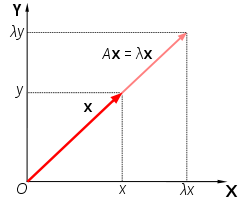Matrix A acts by stretching the vector x, not changing its direction, so x is an eigenvector of A.

Eigenvectors and eigenvalues depend on the concepts of vectors and linear transformations. In the most elementary case, vectors can be thought of as arrows that have both length (or magnitude) and direction. Once a set of Cartesian coordinates is established, a vector can be described relative to that set of coordinates by a sequence of numbers. A linear transformation can be described by a square matrix. For example, in the standard coordinates of n-dimensional space, a vector can be written$\mathbf x = \begin{bmatrix} x_1 \\ x_2 \\ \vdots \\ x_n \end{bmatrix}.$

A matrix can be written$A = \begin{bmatrix} a_{1,1} & a_{1,2} & \ldots & a_{1,n} \\ a_{2,1} & a_{2,2} & \ldots & a_{2,n} \\ \vdots & \vdots & \ddots & \vdots \\ a_{n,1} & a_{n,2} & \ldots & a_{n,n} \\ \end{bmatrix}.$

Here n is some fixed natural number.

Usually, the multiplication of a vector x by a square matrix A changes both the magnitude and the direction of the vector it acts on—but in the special case where it changes only the scale (magnitude) of the vector and leaves the direction unchanged, or switches the vector to the opposite direction, that vector is called an eigenvector of that matrix. (The term "eigenvector" is meaningless except in relation to some particular matrix.) When multiplied by a matrix, each eigenvector of that matrix changes its magnitude by a factor, called the eigenvalue corresponding to that eigenvector.

The vector x is an eigenvector of the matrix A with eigenvalue λ (lambda) if the following equation holds:$\mathbf {Ax} = \lambda\mathbf x.$

This equation can be interpreted geometrically as follows: a vector x is an eigenvector if multiplication by A stretches, shrinks, leaves unchanged, flips (points in the opposite direction), flips and stretches, or flips and shrinks x. If the eigenvalue λ > 1, x is stretched by this factor. If λ = 1, the vector x is not affected at all by multiplication by A. If 0 < λ < 1, x is shrunk (or compressed). The case λ = 0 means that x shrinks to a point (represented by the origin), meaning that x is in the kernel of the linear map given by A. If λ < 0 then x flips and points in the opposite direction as well as being scaled by a factor equal to the absolute value of λ.

As a special case, the identity matrix I is the matrix that leaves all vectors unchanged:$I \mathbf x = 1 \mathbf x = \mathbf x. \,$

Every non-zero vector x is an eigenvector of the identity matrix with eigenvalue 1.

### Example

For the matrix A$A = \begin{bmatrix} 2 & 1\\1 & 2 \end{bmatrix}.$

the vector$\mathbf x = \begin{bmatrix} 3 \\ -3 \end{bmatrix}$

is an eigenvector with eigenvalue 1. Indeed,$A \mathbf x = \begin{bmatrix} 2 & 1\\1 & 2 \end{bmatrix} \begin{bmatrix} 3 \\ -3 \end{bmatrix} = \begin{bmatrix} 2 \cdot 3 + 1 \cdot (-3) \\ 1 \cdot 3 + 2 \cdot (-3) \end{bmatrix} = \begin{bmatrix} 3 \\ -3 \end{bmatrix} = 1 \cdot \begin{bmatrix} 3 \\ -3 \end{bmatrix}.$

On the other hand the vector$\mathbf x = \begin{bmatrix} 0 \\ 1 \end{bmatrix}$

is not an eigenvector, since$\begin{bmatrix} 2 & 1\\1 & 2 \end{bmatrix} \begin{bmatrix} 0 \\ 1 \end{bmatrix} = \begin{bmatrix} 2 \cdot 0 + 1 \cdot 1 \\ 1 \cdot 0 + 2 \cdot 1 \end{bmatrix} = \begin{bmatrix} 1 \\ 2 \end{bmatrix}.$

and this vector is not a multiple of the original vector x.

### Formal definition

In abstract mathematics, a more general definition is given:

Let V be any vector space, let x be a vector in that vector space, and let T be a linear transformation mapping V into V. Then x is an eigenvector of T with eigenvalue λ if the following equation holds:$\mathbf {Tx} = \lambda\mathbf x.$

This equation is called the eigenvalue equation. Note that Tx means T of x, the action of the transformation T on x, while λx means the product of the number λ times the vector x. Most, but not all  authors also require x to be non-zero. The set of eigenvalues of T is sometimes called the spectrum of T.

## Eigenvalues and eigenvectors of matrices

### Characteristic polynomial

The eigenvalues of A are precisely the solutions λ to the equation$\det(A - \lambda I) = 0\, .$

Here det is the determinant of matrix formed by A - λI and I is the n×n identity matrix. This equation is called the characteristic equation (or, less often, the secular equation) of A. For example, if A is the following matrix (a so-called diagonal matrix):$A = \begin{bmatrix} a_{1,1} & 0 & \cdots & 0 \\ 0 & a_{2,2} & \cdots & 0 \\ \vdots & \vdots & \ddots & 0 \\ 0 & 0 & 0 & a_{n,n} \end{bmatrix},$$\det (A-\lambda I) = \det \left(\begin{bmatrix} a_{1,1} & 0 & \cdots & 0 \\ 0 & a_{2,2} & \cdots & 0 \\ \vdots & \vdots & \ddots & 0 \\ 0 & 0 & 0 & a_{n,n} \end{bmatrix} - \lambda \begin{bmatrix} 1 & 0 & \cdots & 0 \\ 0 & 1 & \cdots & 0 \\ \vdots & \vdots & \ddots & 0 \\ 0 & 0 & 0 & 1 \end{bmatrix}\right)$\begin{align} & = \det \begin{bmatrix} a_{1,1} - \lambda & 0 & \cdots & 0 \\ 0 & a_{2,2} - \lambda& \cdots & 0 \\ \vdots & \vdots & \ddots & 0 \\ 0 & 0 & 0 & a_{n,n} - \lambda \end{bmatrix} \\ & = (a_{1,1} - \lambda) (a_{2,2} - \lambda) \cdots (a_{n,n}- \lambda) = 0 \end{align}.

The solutions to this equation are the eigenvalues λi = ai,i (i = 1, ..., n).

Proving the afore-mentioned relation of eigenvalues and solutions of the characteristic equation requires some linear algebra, specifically the notion of linearly independent vectors: briefly, the eigenvalue equation for a matrix A can be expressed as$A \mathbf{x} - \lambda I \mathbf{x} = \mathbf{0} \, ,$

which can be rearranged to$(A - \lambda I) \mathbf{x} = \mathbf{0} \, .$

If there exists an inverse$\displaystyle(A - \lambda I)^{-1},$

then both sides can be left-multiplied by it, to obtain x = 0. Therefore, if λ is such that A − λI is invertible, λ cannot be an eigenvalue. It can be shown that the converse holds, too: if A − λI is not invertible, λ is an eigenvalue. A criterion from linear algebra states that a matrix (here: A − λI) is non-invertible if and only if its determinant is zero, thus leading to the characteristic equation.

The left-hand side of this equation can be seen (using Leibniz' rule for the determinant) to be a polynomial function in λ, whose coefficients depend on the entries of A. This polynomial is called the characteristic polynomial. Its degree is n, that is to say, the highest power of λ occurring in this polynomial is λn. At least for small matrices, the solutions of the characteristic equation (hence, the eigenvalues of A) can be found directly. Moreover, it is important for theoretical purposes, such as the Cayley–Hamilton theorem. It also shows that any n×n matrix has at most n eigenvalues. However, the characteristic equation need not have n distinct solutions. In other words, there may be strictly less than n distinct eigenvalues. This happens for the matrix describing the shear mapping discussed below.

If the matrix has real entries, the coefficients of the characteristic polynomial are all real. However, the roots are not necessarily real; they may include complex numbers with a non-zero imaginary component. For example, a 2×2 matrix describing a 45° rotation will not leave any non-zero vector pointing in the same direction. However, there is at least one complex number λ solving the characteristic equation, even if the entries of the matrix A are complex numbers to begin with. (This existence of such a solution is known as the fundamental theorem of algebra.) For a complex eigenvalue, the corresponding eigenvectors also have complex components.

### Eigenspace

If x is an eigenvector of the matrix A with eigenvalue λ, then any scalar multiple αx is also an eigenvector of A with the same eigenvalue, since Ax) = αAx = αλx = λ(αx). More generally, any non-zero linear combination of eigenvectors that share the same eigenvalue λ, will itself be an eigenvector with eigenvalue λ. Together with the zero vector, the eigenvectors of A with the same eigenvalue form a linear subspace of the vector space called an eigenspace, Eλ. In case of dim(Eλ) = 1, it is called an eigenline and λ is called a scaling factor.

Diagonalizable matrices can be decomposed into a direct sum of eigenspaces, as per the eigendecomposition of a matrix. If a matrix is not diagonalizable, then it is called defective, and, while it cannot be decomposed into eigenspaces, it can be decomposed into the more general concept of generalized eigenspaces, as discussed here.

### Algebraic and geometric multiplicities

Given an n×n matrix A and an eigenvalue λi of this matrix, there are two numbers measuring, roughly speaking, the number of eigenvectors belonging to λi. They are called multiplicities: the algebraic multiplicity of an eigenvalue is defined as the multiplicity of the corresponding root of the characteristic polynomial. The geometric multiplicity of an eigenvalue is defined as the dimension of the associated eigenspace, i.e. number of linearly independent eigenvectors with that eigenvalue. Both algebraic and geometric multiplicity are integers between (including) 1 and n. The algebraic multiplicity ni and geometric multiplicity mi may or may not be equal, but we always have mini. The simplest case is of course when mi = ni = 1. The total number of linearly independent eigenvectors, Nx, is given by summing the geometric multiplicities$\sum\limits_{i=1}^{N_{\lambda}}{m_i} =N_{\mathbf{x}}.$

Over a complex vector space, the sum of the algebraic multiplicities will equal the dimension of the vector space, but the sum of the geometric multiplicities may be smaller. In this case, it is possible that there may not be sufficient eigenvectors to span the entire space – more formally, there is no basis of eigenvectors (an eigenbasis). A matrix is diagonalizable by a suitable choice of coordinates if and only if there is an eigenbasis; if a matrix is not diagonalizable, it is said to be defective. For defective matrices, the notion of eigenvector can be generalized to generalized eigenvectors, and over an algebraically closed field a basis of generalized eigenvectors always exists, as follows from Jordan form.

The eigenvectors corresponding to different eigenvalues are linearly independent, meaning, in particular, that in an n-dimensional space the linear transformation A cannot have more than n eigenvalues (or eigenspaces). All defective matrices have fewer than n distinct eigenvalues, but not all matrices with fewer than n distinct eigenvalues are defective – for example, the identity matrix is diagonalizable (and indeed diagonal in any basis), but only has the eigenvalue 1.

Given an ordered choice of linearly independent eigenvectors, especially an eigenbasis, they can be indexed by eigenvalues, i.e. using a double index, with xi,j being the j th eigenvector for the i th eigenvalue. The eigenvectors can also be indexed using the simpler notation of a single index xk, with k = 1, 2, ... , Nx.

### Worked example

These concepts are explained for the matrix$A = \begin{bmatrix} 2 & 1\\1 & 2 \end{bmatrix}.$

The characteristic equation of this matrix reads$\det (A - \lambda I) = \det\begin{bmatrix} 2-\lambda & 1\\1 & 2-\lambda \end{bmatrix} = 0 \,.$

Calculating the determinant, this yields the quadratic equation$\lambda^2 - 4 \lambda + 3 = 0, \,$

whose solutions (also called roots) are λ = 1 and λ = 3. The eigenvectors for the eigenvalue λ = 3 are determined by using the eigenvalue equation, which in this case reads$\begin{bmatrix} 2 & 1\\1 & 2 \end{bmatrix}\begin{bmatrix}x\\y\end{bmatrix} = 3 \begin{bmatrix}x\\y\end{bmatrix}.$

The juxtaposition at the left hand side denotes matrix multiplication. Spelling this out, this equation comparing two vectors is tantamount to a system of the following two linear equations:$2x+y=3x\,$$x+2y=3y.\,$

Both equations reduce to the single linear equation x = y. That is to say, any vector of the form (x, y) with y = x is an eigenvector to the eigenvalue λ = 3. However, the vector (0, 0) is excluded. A similar calculation shows that the eigenvectors corresponding to the eigenvalue λ = 1, are given by non-zero vectors (x, y) such that y = −x. For example, an eigenvector corresponding to λ = 1, is$\begin{bmatrix}-1\\1\end{bmatrix}$ whereas an eigenvector corresponding to λ = 3, is$\begin{bmatrix}1\\1\end{bmatrix}$. These vectors, placed as columns in a matrix, may be used to create a diagonalizable matrix.

### Eigendecomposition

The spectral theorem for matrices can be stated as follows. Let A be a square n × n matrix. Let q1 ... qk be an eigenvector basis, i.e. an indexed set of k linearly independent eigenvectors, where k is the dimension of the space spanned by the eigenvectors of A. If k = n, then A can be written$\mathbf{A}=\mathbf{Q}\mathbf{\Lambda}\mathbf{Q}^{-1}$

where Q is the square n × n matrix whose i-th column is the basis eigenvector qi of A and Λ is the diagonal matrix whose diagonal elements are the corresponding eigenvalues, i.e. Λii = λi.

### Further properties

Let A be an n×n matrix with eigenvalues λi,$i=1,2,\dots,n$. Then$\operatorname{tr}(A) = \sum \lambda_i= \lambda_1+ \lambda_2 +\cdots+ \lambda_n$.$\operatorname{det}(A) = \prod \lambda_i=\lambda_1\lambda_2\cdots\lambda_n$.
• Eigenvalues of Ak are$\lambda_1^k,\dots,\lambda_n^k$
These first three results follow by putting the matrix in upper-triangular form, in which case the eigenvalues are on the diagonal and the trace and determinant are respectively the sum and product of the diagonal.
• If A = AH, i.e., A is Hermitian, every eigenvalue is real.

### Examples in the plane

The following table presents some example transformations in the plane along with their 2×2 matrices, eigenvalues, and eigenvectors.

 horizontal shear scaling unequal scaling counterclockwise rotation by φ illustration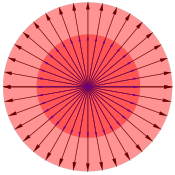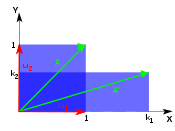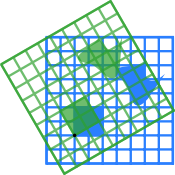matrix$\begin{bmatrix}1 & k\\ 0 & 1\end{bmatrix}$$\begin{bmatrix}k & 0\\0 & k\end{bmatrix}$$\begin{bmatrix}k_1 & 0\\0 & k_2\end{bmatrix}$$\begin{bmatrix} \cos \varphi & -\sin \varphi \\ \sin \varphi & \cos \varphi \end{bmatrix}$ characteristic equation λ2 − 2λ+1 = (1 − λ)2 = 0 λ2 − 2λk + k2 = (λ − k)2 = 0 (λ − k1)(λ − k2) = 0 λ2 − 2λ cos φ + 1 = 0 eigenvalues λi λ1=1 λ1=k λ1 = k1, λ2 = k2 λ1,2 = cos φ ± i sin φ = e ± iφ algebraic and geometric multiplicities n1 = 2, m1 = 1 n1 = 2, m1 = 2 n1 = m1 = 1, n2 = m2 = 1 n1 = m1 = 1, n2 = m2 = 1 eigenvectors$\mathbf u_1 = (1, 0)$$\mathbf u_1 = (1, 0), \mathbf u_2 = (0,1)$$\mathbf u_1 = (1, 0), \mathbf u_2 = (0,1)$$\mathbf u_1 = \begin{bmatrix}1\\-i\end{bmatrix}, \mathbf u_2 = \begin{bmatrix}1\\i\end{bmatrix}.$

#### Shear

Shear in the plane is a transformation where all points along a given line remain fixed while other points are shifted parallel to that line by a distance proportional to their perpendicular distance from the line. In the horizontal shear depicted above, a point P of the plane moves parallel to the x-axis to the place P' so that its coordinate y does not change while the x coordinate increments to become x' = x + k y, where k is called the shear factor. The shear angle φ is determined by k = cot φ.

Repeatedly applying the shear transformation changes the direction of any vector in the plane closer and closer to the direction of the eigenvector.

#### Uniform scaling and reflection

Multiplying every vector with a constant real number k is represented by the diagonal matrix whose entries on the diagonal are all equal to k. Mechanically, this corresponds to stretching a rubber sheet equally in all directions such as a small area of the surface of an inflating balloon. All vectors originating at origin (i.e., the fixed point on the balloon surface) are stretched equally with the same scaling factor k while preserving its original direction. Thus, every non-zero vector is an eigenvector with eigenvalue k. Whether the transformation is stretching (elongation, extension, inflation), or shrinking (compression, deflation) depends on the scaling factor: if k > 1, it is stretching; if 0 < k < 1, it is shrinking. Negative values of k correspond to a reversal of direction, followed by a stretch or a shrink, depending on the absolute value of k.

#### Unequal scaling

For a slightly more complicated example, consider a sheet that is stretched unequally in two perpendicular directions along the coordinate axes, or, similarly, stretched in one direction, and shrunk in the other direction. In this case, there are two different scaling factors: k1 for the scaling in direction x, and k2 for the scaling in direction y. If a given eigenvalue is greater than 1, the vectors are stretched in the direction of the corresponding eigenvector; if less than 1, they are shrunken in that direction. Negative eigenvalues correspond to reflections followed by a stretch or shrink. In general, matrices that are diagonalizable over the real numbers represent scalings and reflections: the eigenvalues represent the scaling factors (and appear as the diagonal terms), and the eigenvectors are the directions of the scalings.

The figure shows the case where k1 > 1 and 1 > k2 > 0. The rubber sheet is stretched along the x axis and simultaneously shrunk along the y axis. After repeatedly applying this transformation of stretching/shrinking many times, almost any vector on the surface of the rubber sheet will be oriented closer and closer to the direction of the x axis (the direction of stretching). The exceptions are vectors along the y-axis, which will gradually shrink away to nothing.

#### Rotation

A rotation in a plane is a transformation that describes motion of a vector, plane, coordinates, etc., around a fixed point. Clearly, for rotations other than through 0° and 180°, every vector in the real plane will have its direction changed, and thus there cannot be any eigenvectors. But this is not necessarily true if we consider the same matrix over a complex vector space. The characteristic equation is a quadratic equation with discriminant D = 4 (cos2 φ − 1) = − 4 sin2 φ, which is a negative number whenever φ is not equal to a multiple of 180°. A rotation of 0°, 360°, … is just the identity transformation (a uniform scaling by +1), while a rotation of 180°, 540°, …, is a reflection (uniform scaling by -1). Otherwise, as expected, there are no real eigenvalues or eigenvectors for rotation in the plane. Instead, the eigenvalues are complex numbers in general. Although not diagonalizable over the reals, the rotation matrix is diagonalizable over the complex numbers, and again the eigenvalues appear on the diagonal. Thus rotation matrices acting on complex spaces can be thought of as scaling matrices, with complex scaling factors.

## Calculation

The complexity of the problem for finding roots/eigenvalues of the characteristic polynomial increases rapidly with increasing the degree of the polynomial (the dimension of the vector space). There are exact solutions for dimensions below 5, but for dimensions greater than or equal to 5 there are generally no exact solutions and one has to resort to numerical methods to find them approximately. (In fact, since the roots of any polynomial can be expressed as eigenvalues of a companion matrix, the Abel–Ruffini theorem implies that there is no general algebraic solution for eigenvalues of 5×5 or larger matrices: any general eigenvalue algorithm is necessarily approximate, although in practice one can obtain any desired accuracy.) Worse, any computational procedure that starts by computing the coefficients of the characteristic polynomial can be very inaccurate in the presence of round-off error, because the roots of a polynomial are an extremely sensitive function of the coefficients (see Wilkinson's polynomial). Efficient, accurate methods to compute eigenvalues and eigenvectors of arbitrary matrices were not known until the advent of the QR algorithm in 1961.  Besides, combining Householder transformation with LU decomposition can get better convergence than QR algorithm. For large Hermitian sparse matrices, the Lanczos algorithm is one example of an efficient iterative method to compute eigenvalues and eigenvectors, among several other possibilities.

## History

Eigenvalues are often introduced in the context of linear algebra or matrix theory. Historically, however, they arose in the study of quadratic forms and differential equations.

Euler studied the rotational motion of a rigid body and discovered the importance of the principal axes. Lagrange realized that the principal axes are the eigenvectors of the inertia matrix. In the early 19th century, Cauchy saw how their work could be used to classify the quadric surfaces, and generalized it to arbitrary dimensions. Cauchy also coined the term racine caractéristique (characteristic root) for what is now called eigenvalue; his term survives in characteristic equation.

Fourier used the work of Laplace and Lagrange to solve the heat equation by separation of variables in his famous 1822 book Théorie analytique de la chaleur. Sturm developed Fourier's ideas further and brought them to the attention of Cauchy, who combined them with his own ideas and arrived at the fact that real symmetric matrices have real eigenvalues. This was extended by Hermite in 1855 to what are now called Hermitian matrices. Around the same time, Brioschi proved that the eigenvalues of orthogonal matrices lie on the unit circle, and Clebsch found the corresponding result for skew-symmetric matrices. Finally, Weierstrass clarified an important aspect in the stability theory started by Laplace by realizing that defective matrices can cause instability.

In the meantime, Liouville studied eigenvalue problems similar to those of Sturm; the discipline that grew out of their work is now called Sturm–Liouville theory. Schwarz studied the first eigenvalue of Laplace's equation on general domains towards the end of the 19th century, while Poincaré studied Poisson's equation a few years later.

At the start of the 20th century, Hilbert studied the eigenvalues of integral operators by viewing the operators as infinite matrices. He was the first to use the German word eigen to denote eigenvalues and eigenvectors in 1904, though he may have been following a related usage by Helmholtz. For some time, the standard term in English was "proper value", but the more distinctive term "eigenvalue" is standard today.

The first numerical algorithm for computing eigenvalues and eigenvectors appeared in 1929, when Von Mises published the power method. One of the most popular methods today, the QR algorithm, was proposed independently by John G.F. Francis and Vera Kublanovskaya in 1961.

## Generalizations

### Left and right eigenvectors

The word eigenvector formally refers to the right eigenvector xR. It is defined by the above eigenvalue equation$A x_R = \lambda_R x_R,\$

and is the most commonly used eigenvector. However, the left eigenvector xL exists as well, and is defined by$x_L A = \lambda_L x_L. \$

### Infinite-dimensional spaces and spectral theory

If the vector space is an infinite dimensional Banach space, the notion of eigenvalues can be generalized to the concept of spectrum. The spectrum is the set of scalars λ for which (T − λI)−1 is not defined; that is, such that T − λI has no bounded inverse.

Clearly if λ is an eigenvalue of T, λ is in the spectrum of T. In general, the converse is not true. There are operators on Hilbert or Banach spaces that have no eigenvectors at all. This can be seen in the following example. The bilateral shift on the Hilbert space 2(Z) (that is, the space of all sequences of scalars … a−1, a0, a1, a2, … such that$\cdots + |a_{-1}|^2 + |a_0|^2 + |a_1|^2 + |a_2|^2 + \cdots \,$

converges) has no eigenvalue but does have spectral values.

In infinite-dimensional spaces, the spectrum of a bounded operator is always nonempty. This is also true for an unbounded self adjoint operator. Via its spectral measures, the spectrum of any self adjoint operator, bounded or otherwise, can be decomposed into absolutely continuous, pure point, and singular parts. (See Decomposition of spectrum.)

The hydrogen atom is an example where both types of spectra appear. The eigenfunctions of the hydrogen atom Hamiltonian are called eigenstates and are grouped into two categories. The bound states of the hydrogen atom correspond to the discrete part of the spectrum (they have a discrete set of eigenvalues that can be computed by Rydberg formula) while the ionization processes are described by the continuous part (the energy of the collision/ionization is not quantized).

#### Eigenfunctions

A common example of such maps on infinite dimensional spaces are the action of differential operators on function spaces. As an example, on the space of infinitely differentiable functions, the process of differentiation defines a linear operator since$\displaystyle\frac{d}{dt}(af+bg) = a \frac{df}{dt} + b \frac{dg}{dt},$

where f(t) and g(t) are differentiable functions, and a and b are constants.

The eigenvalue equation for linear differential operators is then a set of one or more differential equations. The eigenvectors are commonly called eigenfunctions. The simplest case is the eigenvalue equation for differentiation of a real valued function by a single real variable. We seek a function (equivalent to an infinite-dimensional vector) that, when differentiated, yields a constant times the original function. In this case, the eigenvalue equation becomes the linear differential equation$\displaystyle\frac{d}{dx} f(x) = \lambda f(x).$

Here λ is the eigenvalue associated with the function, f(x). This eigenvalue equation has a solution for any value of λ. If λ is zero, the solution is$f(x) = A,\,$

where A is any constant; if λ is non-zero, the solution is the exponential function$f(x) = Ae^{\lambda x}.\$

If we expand our horizons to complex valued functions, the value of λ can be any complex number. The spectrum of d/dt is therefore the whole complex plane. This is an example of a continuous spectrum.

##### Waves on a stringThe shape of a standing wave in a string fixed at its boundaries is an example of an eigenfunction of a differential operator. The admittable eigenvalues are governed by the length of the string and determine the frequency of oscillation.

The displacement, h(x,t), of a stressed rope fixed at both ends, like the vibrating strings of a string instrument, satisfies the wave equation$\frac{\partial^2 h}{\partial t^2} = c^2\frac{\partial^2 h}{\partial x^2},$

which is a linear partial differential equation, where c is the constant wave speed. The normal method of solving such an equation is separation of variables. If we assume that h can be written as the product of the form X(x)T(t), we can form a pair of ordinary differential equations:$X''=-\frac{\omega^2}{c^2}X$ and$T''=-\omega^2 T.\$

Each of these is an eigenvalue equation (the unfamiliar form of the eigenvalue is chosen merely for convenience). For any values of the eigenvalues, the eigenfunctions are given by$X = \sin \left(\frac{\omega x}{c} + \phi \right)\$ and$T = \sin(\omega t + \psi).\$

If we impose boundary conditions (that the ends of the string are fixed with X(x) = 0 at x = 0 and x = L, for example) we can constrain the eigenvalues. For those boundary conditions, we find$\sin(\phi) = 0\$, and so the phase angle$\phi=0\$

and$\sin\left(\frac{\omega L}{c}\right) = 0.\$

Thus, the constant ω is constrained to take one of the values$\omega_n = \frac{nc\pi}{L}$, where n is any integer. Thus the clamped string supports a family of standing waves of the form$h(x,t) = \sin(n\pi x/L)\sin(\omega_n t).\$

From the point of view of our musical instrument, the frequency$\omega_n\$ is the frequency of the nth harmonic, which is called the (n-1)st overtone.

### Associative algebras and representation theory

More algebraically, rather than generalizing the vector space to an infinite dimensional space, one can generalize the algebraic object that is acting on the space, replacing a single operator acting on a vector space with an algebra representation – an associative algebra acting on a module. The study of such actions is the field of representation theory. To understand these representations, one breaks them into indecomposable representations, and, if possible, into irreducible representations; these correspond respectively to generalized eigenspaces and eigenspaces, or rather the indecomposable and irreducible components of these. While a single operator on a vector space can be understood in terms of eigenvectors – 1-dimensional invariant subspaces – in general in representation theory the building blocks (the irreducible representations) are higher-dimensional.

A closer analog of eigenvalues is given by the notion of a weight, with the analogs of eigenvectors and eigenspaces being weight vectors and weight spaces. For an associative algebra A over a field F, the analog of an eigenvalue is a one-dimensional representation$\lambda \colon A \to \mathbf{F}$ (a map of algebras; a linear functional that is also multiplicative), called the weight, rather than a single scalar. A map of algebras is used because if a vector is an eigenvector for two elements of an algebra, then it is also an eigenvector for any linear combination of these, and the eigenvalue is the corresponding linear combination of the eigenvalues, and likewise for multiplication. This is related to the classical eigenvalue as follows: a single operator T corresponds to the algebra F[T] (the polynomials in T), and a map of algebras$\mathbf{F}[T] \to \mathbf{F}$ is determined by its value on the generator T; this value is the eigenvalue. A vector v on which the algebra acts by this weight (i.e., by scalar multiplication, with the scalar determined by the weight) is called a weight vector, and other concepts generalize similarly. The generalization of a diagonalizable matrix (having an eigenbasis) is a weight module.

Because a weight is a map to a field, which is commutative, the map factors through the abelianization of the algebra A – equivalently, it vanishes on the derived algebra – in terms of matrices, if v is a common eigenvector of operators T and U, then TUv = UTv (because in both cases it is just multiplication by scalars), so common eigenvectors of an algebra must be in the set on which the algebra acts commutatively (which is annihilated by the derived algebra). Thus of central interest are the free commutative algebras, namely the polynomial algebras. In this particularly simple and important case of the polynomial algebra$\mathbf{F}[T_1,\dots,T_k]$ in a set of commuting matrices, a weight vector of this algebra is a simultaneous eigenvector of the matrices, while a weight of this algebra is simply a k-tuple of scalars$\lambda = (\lambda_1,\dots,\lambda_k)$ corresponding to the eigenvalue of each matrix, and hence geometrically to a point in k-space. These weights – in particularly their geometry – are of central importance in understanding the representation theory of Lie algebras, specifically the finite-dimensional representations of semisimple Lie algebras.

As an application of this geometry, given an algebra that is a quotient of a polynomial algebra on k generators, it corresponds geometrically to an algebraic variety in k-dimensional space, and the weight must fall on the variety – i.e., it satisfies defining equations for the variety. This generalizes the fact that eigenvalues satisfy the characteristic polynomial of a matrix in one variable.

## Applications

### Schrödinger equation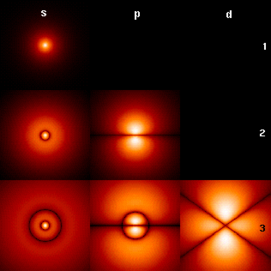The wavefunctions associated with the bound states of an electron in a hydrogen atom can be seen as the eigenvectors of the hydrogen atom Hamiltonian as well as of the angular momentum operator. They are associated with eigenvalues interpreted as their energies (increasing downward: n=1,2,3,...) and angular momentum (increasing across: s, p, d,...). The illustration shows the square of the absolute value of the wavefunctions. Brighter areas correspond to higher probability density for a position measurement. The center of each figure is the atomic nucleus, a proton.

An example of an eigenvalue equation where the transformation T is represented in terms of a differential operator is the time-independent Schrödinger equation in quantum mechanics:$H\psi_E = E\psi_E \,$

where H, the Hamiltonian, is a second-order differential operator and ψE, the wavefunction, is one of its eigenfunctions corresponding to the eigenvalue E, interpreted as its energy.

However, in the case where one is interested only in the bound state solutions of the Schrödinger equation, one looks for ψE within the space of square integrable functions. Since this space is a Hilbert space with a well-defined scalar product, one can introduce a basis set in which ψE and H can be represented as a one-dimensional array and a matrix respectively. This allows one to represent the Schrödinger equation in a matrix form.

Bra-ket notation is often used in this context. A vector, which represents a state of the system, in the Hilbert space of square integrable functions is represented by$|\Psi_E\rangle$. In this notation, the Schrödinger equation is:$H|\Psi_E\rangle = E|\Psi_E\rangle$

where$|\Psi_E\rangle$ is an eigenstate of H. It is a self adjoint operator, the infinite dimensional analog of Hermitian matrices (see Observable). As in the matrix case, in the equation above$H|\Psi_E\rangle$ is understood to be the vector obtained by application of the transformation H to$|\Psi_E\rangle$.

### Molecular orbitals

In quantum mechanics, and in particular in atomic and molecular physics, within the Hartree–Fock theory, the atomic and molecular orbitals can be defined by the eigenvectors of the Fock operator. The corresponding eigenvalues are interpreted as ionization potentials via Koopmans' theorem. In this case, the term eigenvector is used in a somewhat more general meaning, since the Fock operator is explicitly dependent on the orbitals and their eigenvalues. If one wants to underline this aspect one speaks of nonlinear eigenvalue problem. Such equations are usually solved by an iteration procedure, called in this case self-consistent field method. In quantum chemistry, one often represents the Hartree–Fock equation in a non-orthogonal basis set. This particular representation is a generalized eigenvalue problem called Roothaan equations.

### Geology and glaciology

In geology, especially in the study of glacial till, eigenvectors and eigenvalues are used as a method by which a mass of information of a clast fabric's constituents' orientation and dip can be summarized in a 3-D space by six numbers. In the field, a geologist may collect such data for hundreds or thousands of clasts in a soil sample, which can only be compared graphically such as in a Tri-Plot (Sneed and Folk) diagram, or as a Stereonet on a Wulff Net. The output for the orientation tensor is in the three orthogonal (perpendicular) axes of space. Eigenvectors output from programs such as Stereo32  are in the order E1 ≥ E2 ≥ E3, with E1 being the primary orientation of clast orientation/dip, E2 being the secondary and E3 being the tertiary, in terms of strength. The clast orientation is defined as the eigenvector, on a compass rose of 360°. Dip is measured as the eigenvalue, the modulus of the tensor: this is valued from 0° (no dip) to 90° (vertical). The relative values of E1, E2, and E3 are dictated by the nature of the sediment's fabric. If E1 = E2 = E3, the fabric is said to be isotropic. If E1 = E2 > E3 the fabric is planar. If E1 > E2 > E3 the fabric is linear. See 'A Practical Guide to the Study of Glacial Sediments' by Benn & Evans, 2004.

### Principal components analysis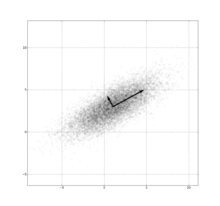PCA of the multivariate Gaussian distribution centered at (1,3) with a standard deviation of 3 in roughly the (0.878, 0.478) direction and of 1 in the orthogonal direction. The vectors shown are unit eigenvectors of the (symmetric, positive-semidefinite) covariance matrix scaled by the square root of the corresponding eigenvalue. (Just as in the one-dimensional case, the square root is taken because the standard deviation is more readily visualized than the variance.

The eigendecomposition of a symmetric positive semidefinite (PSD) matrix yields an orthogonal basis of eigenvectors, each of which has a nonnegative eigenvalue. The orthogonal decomposition of a PSD matrix is used in multivariate analysis, where the sample covariance matrices are PSD. This orthogonal decomposition is called principal components analysis (PCA) in statistics. PCA studies linear relations among variables. PCA is performed on the covariance matrix or the correlation matrix (in which each variable is scaled to have its sample variance equal to one). For the covariance or correlation matrix, the eigenvectors correspond to principal components and the eigenvalues to the variance explained by the principal components. Principal component analysis of the correlation matrix provides an orthonormal eigen-basis for the space of the observed data: In this basis, the largest eigenvalues correspond to the principal-components that are associated with most of the covariability among a number of observed data.

Principal component analysis is used to study large data sets, such as those encountered in data mining, chemical research, psychology, and in marketing. PCA is popular especially in psychology, in the field of psychometrics. In Q-methodology, the eigenvalues of the correlation matrix determine the Q-methodologist's judgment of practical significance (which differs from the statistical significance of hypothesis testing): The factors with eigenvalues greater than 1.00 are considered practically significant, that is, as explaining an important amount of the variability in the data, while eigenvalues less than 1.00 are considered practically insignificant, as explaining only a negligible portion of the data variability. More generally, principal component analysis can be used as a method of factor analysis in structural equation modeling.

### Vibration analysis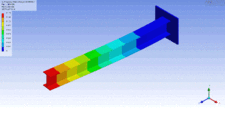1st lateral bending (See vibration for more types of vibration)

Eigenvalue problems occur naturally in the vibration analysis of mechanical structures with many degrees of freedom. The eigenvalues are used to determine the natural frequencies (or eigenfrequencies) of vibration, and the eigenvectors determine the shapes of these vibrational modes. In particular, undamped vibration is governed by$m\ddot x + kx = 0$

or$m\ddot x = -k x$

that is, acceleration is proportional to position (i.e., we expect x to be sinusoidal in time). In n dimensions, m becomes a mass matrix and k a stiffness matrix. Admissible solutions are then a linear combination of solutions to the generalized eigenvalue problem

kx = ω2mx

where ω2 is the eigenvalue and ω is the angular frequency. Note that the principal vibration modes are different from the principal compliance modes, which are the eigenvectors of k alone. Furthermore, damped vibration, governed by$m\ddot x + c \dot x + kx = 0$

2m + ωc + k)x = 0.

This can be reduced to a generalized eigenvalue problem by clever algebra at the cost of solving a larger system.

The orthogonality properties of the eigenvectors allows decoupling of the differential equations so that the system can be represented as linear summation of the eigenvectors. The eigenvalue problem of complex structures is often solved using finite element analysis, but neatly generalize the solution to scalar-valued vibration problems.

### Eigenfaces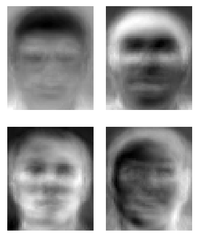Eigenfaces as examples of eigenvectors

In image processing, processed images of faces can be seen as vectors whose components are the brightnesses of each pixel. The dimension of this vector space is the number of pixels. The eigenvectors of the covariance matrix associated with a large set of normalized pictures of faces are called eigenfaces; this is an example of principal components analysis. They are very useful for expressing any face image as a linear combination of some of them. In the facial recognition branch of biometrics, eigenfaces provide a means of applying data compression to faces for identification purposes. Research related to eigen vision systems determining hand gestures has also been made.

Similar to this concept, eigenvoices represent the general direction of variability in human pronunciations of a particular utterance, such as a word in a language. Based on a linear combination of such eigenvoices, a new voice pronunciation of the word can be constructed. These concepts have been found useful in automatic speech recognition systems, for speaker adaptation.

### Tensor of inertia

In mechanics, the eigenvectors of the inertia tensor define the principal axes of a rigid body. The tensor of inertia is a key quantity required to determine the rotation of a rigid body around its center of mass.

### Stress tensor

In solid mechanics, the stress tensor is symmetric and so can be decomposed into a diagonal tensor with the eigenvalues on the diagonal and eigenvectors as a basis. Because it is diagonal, in this orientation, the stress tensor has no shear components; the components it does have are the principal components.

### Eigenvalues of a graph

In spectral graph theory, an eigenvalue of a graph is defined as an eigenvalue of the graph's adjacency matrix A, or (increasingly) of the graph's Laplacian matrix (see also Discrete Laplace operator), which is either TA (sometimes called the Combinatorial Laplacian) or IT−1/2AT−1/2 (sometimes called the Normalized Laplacian), where T is a diagonal matrix with Tv,v equal to the degree of vertex v, and in T−1/2, the vth diagonal entry is deg(v)−1/2. The kth principal eigenvector of a graph is defined as either the eigenvector corresponding to the kth largest or kth smallest eigenvalue of the Laplacian. The first principal eigenvector of the graph is also referred to merely as the principal eigenvector.

The principal eigenvector is used to measure the centrality of its vertices. An example is Google's PageRank algorithm. The principal eigenvector of a modified adjacency matrix of the World Wide Web graph gives the page ranks as its components. This vector corresponds to the stationary distribution of the Markov chain represented by the row-normalized adjacency matrix; however, the adjacency matrix must first be modified to ensure a stationary distribution exists. The second smallest eigenvector can be used to partition the graph into clusters, via spectral clustering. Other methods are also available for clustering.

### Basic reproduction number

See Basic reproduction number

The basic reproduction number (R0) is a fundamental number in the study of how infectious diseases spread. If one infectious person is put into a population of completely susceptible people, then R0 is the average number of people that one infectious person will infect. The generation time of an infection is the time, tG, from one person become infected to the next person becoming infected. In a heterogenous population, the next generation matrix defines how many people in the population will become infected after time tG has passed. R0 is then the largest eigenvalue of the next generation matrix. This result is due to Heesterbeek, at the University of Utrecht.

Wikimedia Foundation. 2010.

### Look at other dictionaries:

• Eigenvalue, eigenvector and eigenspace — In mathematics, given a linear transformation, an Audio|De eigenvector.ogg|eigenvector of that linear transformation is a nonzero vector which, when that transformation is applied to it, changes in length, but not direction. For each eigenvector… …   Wikipedia

• Divide-and-conquer eigenvalue algorithm — Divide and conquer eigenvalue algorithms are a class of eigenvalue algorithms for Hermitian or real symmetric matrices that have recently (circa 1990s) become competitive in terms of stability and efficiency with more traditional algorithms such… …   Wikipedia

• Theorems and definitions in linear algebra — This article collects the main theorems and definitions in linear algebra. Vector spaces A vector space( or linear space) V over a number field² F consists of a set on which two operations (called addition and scalar multiplication, respectively) …   Wikipedia

• Tennessee Governor's Academy for Math and Science — Infobox high school name= [http://tga.tennessee.edu Tennessee Governor s Academy for Math and Science] (TGA) motto=Latin: Veni, Vidi, Duci. English: I came; I saw; I calculated. type=Public Residential established=2007 faculty=Three full time… …   Wikipedia

• Eigendecomposition of a matrix — In the mathematical discipline of linear algebra, eigendecomposition or sometimes spectral decomposition is the factorization of a matrix into a canonical form, whereby the matrix is represented in terms of its eigenvalues and… …   Wikipedia

• Eigenvalue algorithm — In linear algebra, one of the most important problems is designing efficient and stable algorithms for finding the eigenvalues of a matrix. These eigenvalue algorithms may also find eigenvectors. Contents 1 Characteristic polynomial 2 Power… …   Wikipedia

• Principal component analysis — PCA of a multivariate Gaussian distribution centered at (1,3) with a standard deviation of 3 in roughly the (0.878, 0.478) direction and of 1 in the orthogonal direction. The vectors shown are the eigenvectors of the covariance matrix scaled by… …   Wikipedia

• Discrete Fourier transform — Fourier transforms Continuous Fourier transform Fourier series Discrete Fourier transform Discrete time Fourier transform Related transforms In mathematics, the discrete Fourier transform (DFT) is a specific kind of discrete transform, used in… …   Wikipedia

• Diagonalizable matrix — In linear algebra, a square matrix A is called diagonalizable if it is similar to a diagonal matrix, i.e., if there exists an invertible matrix P such that P −1AP is a diagonal matrix. If V is a finite dimensional vector space, then a linear …   Wikipedia

• Jacobi eigenvalue algorithm — The Jacobi eigenvalue algorithm is a numerical procedure for the calculation of all eigenvalues and eigenvectors of a real symmetric matrix. Description Let varphi in mathbb{R}, , 1 le k < l le n and let J(varphi, k, l) denote the n imes n matrix …   Wikipedia